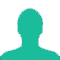# 1.14 expressed as a per cent of 1.9 is:

A. 6%

B. 10%

C. 60%

D. 90%

### Solution(By Examveda Team)

\eqalign{ & {\text{Required}}\,{\text{Percentage}} \cr & = \frac{{ {1.14 \times 100} }}{{1.9}} \cr & = 60\% \cr}

This Question Belongs to Arithmetic Ability >> Percentage

1.1.14÷1.9=0.6
0.6×100
60

2.60%

3.60

Related Questions on Percentage